# Using the law of reflection

When we observe an object in a mirror there are two ways to work out how the light rays from an object focus into the eye of an observer:

1. Use the laws of reflection

or

2. Use ray diagrams and a virtual image

Here we shall discuss method 1, using the laws of reflection.

In the following diagram we will try to find the light rays from an object which travel and focus in the eye of an observer: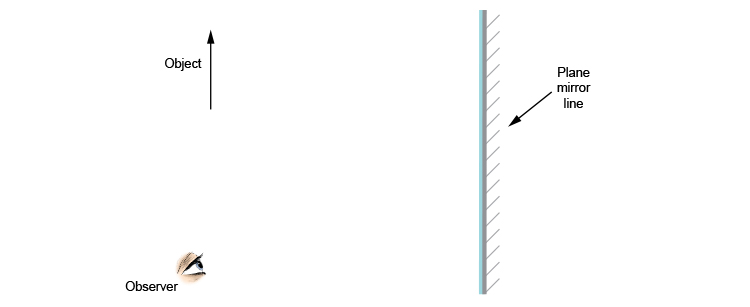Place a protractor with its straight edge along the plane mirror. Use a ruler to extend the protracted lines. Move the protractor up and down until you have the point of the object arrow (incident ray) and the observer (reflected ray) with an equal angle between these rays and the 90° mark on the protractor.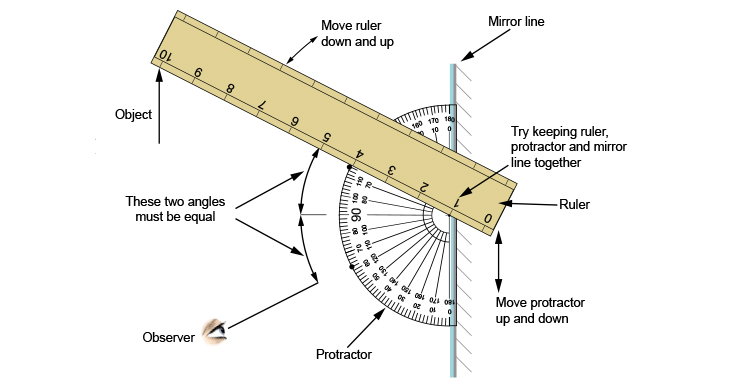As you can see, or hopefully as you have experimented, aligning the ruler, protractor and mirror and trying to work out an equal angle either side of the 90° on the protractor is difficult and time-consuming.

This uses the main law of reflection which states:

The angle between the incident ray and the normal is equal to the angle between the reflected ray and the normal.

When the angle between the incident ray and the normal is equal to the angle between the reflected ray and the normal we can then mark a dot on the outside edge of the protractor where this occurs. You can then draw the incident ray to the mirror and the reflected ray to the observer.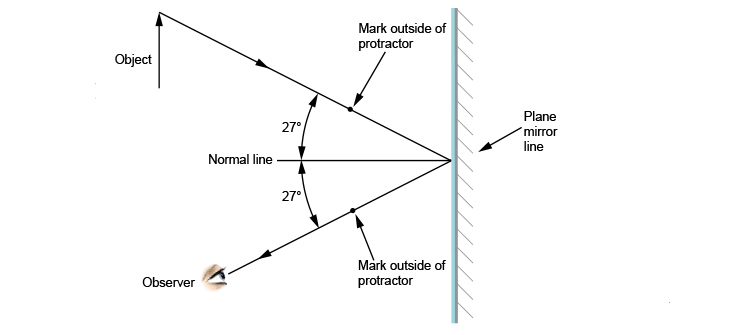Now we have to carry out the same procedure from the bottom of the object to the observer. Again we use the protractor and a ruler.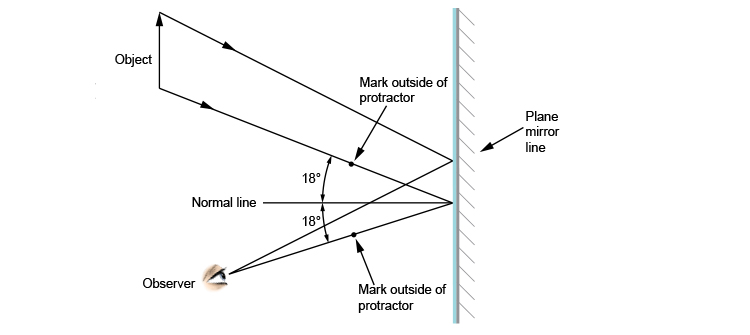Now we have finally worked out how the light rays from an object focus into the eye of an observer.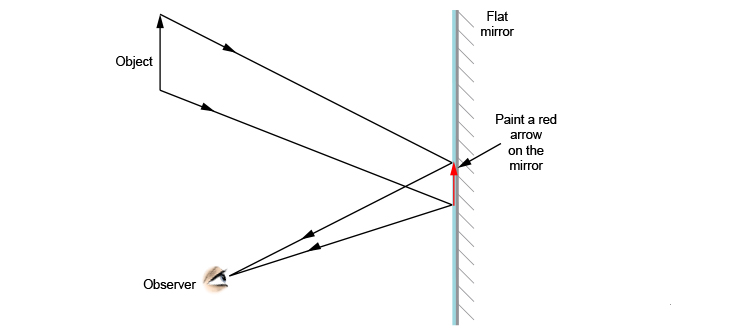If you could ask someone to paint, in red, where the arrow was on the plane mirror it would look like the above diagram.

If you removed the object and left the red arrow you wouldn't know that the object had been removed.

## Conclusion

The above is a very time-consuming method for finding the projected rays from an object. But why bother when there is a much easier method for finding these lines from a flat mirror?

Some very smart scientists set up ray diagram rules and these will be shown in the next section.

NOTE: These rules are all about creating an imaginary (what people call virtual) image behind the mirror.

This method is a lot easier.